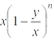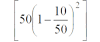# Mixture and Alligation: Concepts and Tricks

In this article, we are going to discuss the rule of mixtures and alligations. Alligation is an important area of quantitative aptitude for various competitive exams. The rule of alligation enables us to find the ratio in which two or more ingredients at the given price must be mixed to produce a mixture of a desired price. This technique can be applied to any topic like mixtures, profit & loss, simple interest, time & distance, percentage, etc.
Rule of Alligation
Alligation is a rule that enables us to find the ratio in which two or more ingredients at the given price must be mixed to produce a mixture of desired price. There are two types of methods used in alligation.
Alligation Method 1:
• It is a modified form of finding the weighted average. If 2 ingredients are mixed in a ratio and the cost price of the unit quantity of the mixture, called the Mean Price is given then,• The above formula can be represented with the help of diagram which is easier to understand.Here 'd' is the cost of dearer ingredient, 'm' is mean price and 'c' is the cost of cheaper ingredient.Example 1: In what ratio must a shopkeeper mix two types of rice worth Rs. 50 kg and Rs. 70 kg, so that the average cost of the mixture is Rs. 65 kg?
Solution: By Rule of alligation,Hence the answer is 5:15 or 1:3.
Alligation Method 2: Repeated Dilution
• This is used to calculate pure quantity left after 'n' number of processes of repeated replacement is done on the pure quantity. Suppose, a container contains 'x' units of a liquid from which 'y' units are taken out and replaced by water. After 'n' operations quantity of pureExample 2: A container contains 50 litres of milk. From this container, 10 litres of milk was taken out and replaced by water. This process is repeated one more time.
How much milk is now left in the container?
1. 24
2. 32
3. 30
4. 36
Solution: Applying the Replacement Method: Amount of milk after 2 operations =Watch Video
Mixture and Alligation: Key Learning
• In this article we learned the techniques of alligations and mixtures. Using this technique many questions, which otherwise have longer solutions can be solved very quickly. This topic when mastered makes many questions related to different areas as sitters.
For any query on this topic, feel free to post in the comment section below.